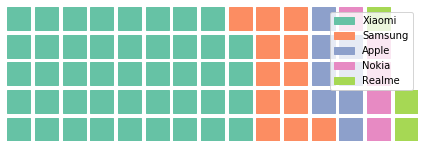# Generate Waffle chart using pyWaffle in Python

• Last Updated : 21 Apr, 2020

A Waffle Chart is a gripping visualization technique that is normally created to display progress towards goals. Where each cell in the Waffle Chart constitutes of `10 X 10` cell grid in which each cell represents one percentage point summing up to total 100%. It is commonly an effective option when you are trying to add interesting visualization features to a visual. Waffle Charts are widely used as an Excel dashboard.

For generating Waffle Chart in Python, modules needed are – matplotlib, pandas and pyWaffle.
To install these packages, run the following commands :

```pip install matplotlib
pip install pandas
pip install pywaffle
```

Below is the implementation:

 `# python program to generate Waffle Chart`` ` `# importing all necessary requirements``import` `pandas as pd``import` `matplotlib.pyplot as plt``from` `pywaffle ``import` `Waffle`` ` `# creation of a dataframe``data ``=``{``'phone'``: [``'Xiaomi'``, ``'Samsung'``,``                 ``'Apple'``, ``'Nokia'``, ``'Realme'``],``       ``'stock'``: [``44``, ``12``, ``8``, ``5``, ``3``]``     ``}`` ` `df ``=` `pd.DataFrame(data)`` ` `# To plot the waffle Chart``fig ``=` `plt.figure(``    ``FigureClass ``=` `Waffle,``    ``rows ``=` `5``,``    ``values ``=` `df.stock,``    ``labels ``=` `list``(df.phone)``)`

Output:The above Waffle Chart has been generated for the data in the DataFrame